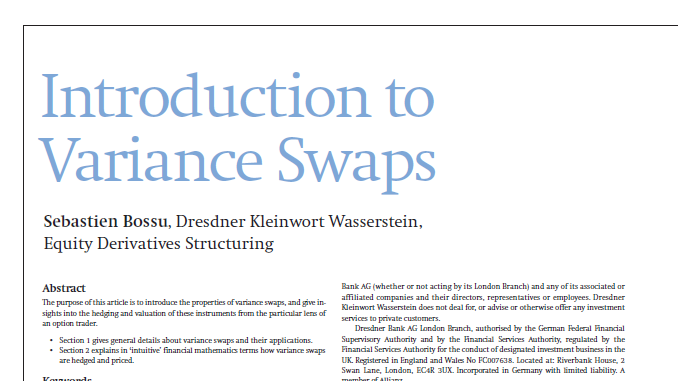# Introduction to Variance Swaps

## Sebastien Bossu introduces the properties of variance swaps, and give insights into the hedging and valuation of these instruments from the particular lens of an option traderThe purpose of this article is to introduce the properties of variance swaps, and give insights into the hedging and valuation of these instruments from the particular lens of an option trader.

Section 1 gives general details about variance swaps and their applications.

Section 2 explains in ‘intuitive’ financial mathematics terms how variance swaps
are hedged and priced.